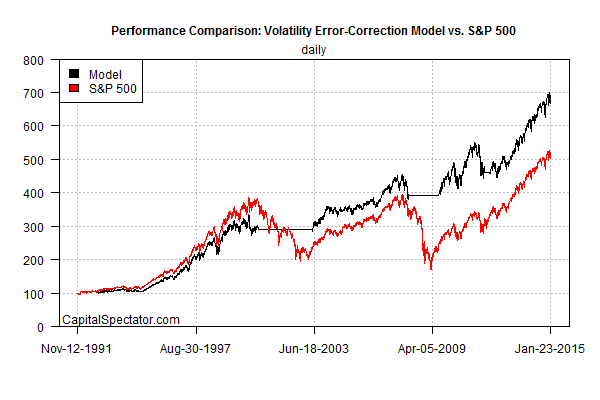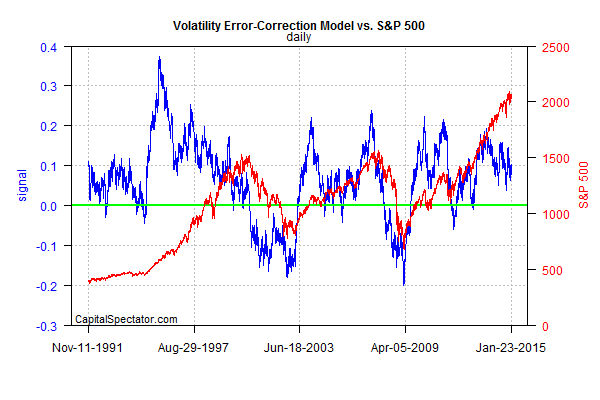# A Momentum-Based Trading Signal With Strategic Value

Traders and investors tend to operate in parallel universes, using different analytical toolkits and looking at markets from radically different perspectives. But sometimes there’s common ground. David Varadi’s recent investigation of what he calls error-adjusted momentum (EAM) to normalize returns by way of volatility is an example. Although he’s focused on developing short-term trading signals, EAM serves double duty as a risk measure for monitoring the potential for severe market corrections. That’s of interest to traders, of course, but it’s a topic of import for long-term investors as well. As such, this signal potentially offers valuable intelligence for managing asset allocation over a medium- to long-term horizon via looking for productive times for rebalancing.

As a preliminary test of EAM’s signal, let’s crunch the numbers and see what a backtest reveals. But first, a quick summary of the logic behind Varadi’s metric. The main idea here is to adjust asset prices/returns by some definition of risk, in this case volatility. Vol is constantly changing, fluctuating between high and low regimes, a dynamic state that has a major influence on market performance. In the equity market, low vol tends to be associated with positive/high returns while high vol is linked with negative/low performance. In a perfect world, we’d forecast vol and adjust the portfolio accordingly. Unfortunately, predicting risk is tricky. It’s easier than trying to forecast return, but it’s still a slippery task. The future’s uncertain, but Varadi’s EAM is an intriguing concept for developing some informed intuition about where vol is headed.

The basic procedure: generate a rolling set of simple return forecasts based on the average performance for the trailing 10-day period. Next, measure the accuracy of the forecasts by calculating the 10-day mean absolute error. Finally, divide the actual daily return by this standard error estimate and then compute the rolling 200-day average for smoothing purposes. The logic, Varadi explains,

is that returns should be weighted more when predictability is high, and conversely weighted less when predictability is low. In this case, the error-adjusted moving average will hopefully be more robust to market noise than a standard moving average.

Although Varadi’s main goal is developing short-term trading intelligence, we can use the signal for context in assessing the risk climate for a given market. As we’ll see, EAM has an encouraging record of flashing warning signals ahead of major market declines. Not surprisingly, that record translates into a market-beating strategy vs. buying and holding the US stock market (S&P 500)

Let’s run a simple backtest in R. First we download the S&P’s daily price history from the close of 1990, and then create a function to implement EAM.

```# load packages
library(TTR)
library(zoo)
library(quantmod)

gspc <-getSymbols('^gspc',from='1990-12-31',auto.assign=FALSE)

eam <-function(x,y,z) { # x=ticker, y=lookback period for forecast z=SMA period
a <-na.omit(ROC(Cl(x),1,"discrete"))
b <-na.omit(SMA(a,y)) # forecast based on "y" trailing period returns
c <-na.omit(Lag(b,k=1)) # lag forecasts by 1 period
d <-na.omit(cbind(c,a)) # combine lagged forecasts with actual returns into one file
e <-as.xts(apply(d,1,diff)) # actual daily return less forecast
f <-to.daily(na.omit(rollapplyr(e,y,function(x) mean(abs(x)))),drop.time=TRUE,OHLC=FALSE) # mean absolute error
g <-cbind(a,f) # combine actual return with MAE into one file
h <-na.omit(a[,1]/g[,2]) # divide actual return by MAE
i <-na.omit(SMA(h,z)) # generate 200-day moving average of adjusted return
j <-na.omit(Lag(ifelse(i >0,1,0))) # lag adjusted return signal by one day for trading analysis
}
```

Now let’s create another function for comparing a portfolio strategy based on EAM’s buy/sell signal vs. simply buying and holding the S&P 500. Note that the EAM strategy here doesn’t include shorting—the portfolio is either holding the S&P or sitting on the sidelines with zero return. As you can see in the chart below, the strategy underperforms for the first decade or so, but does a good job of avoiding the bulk of the 2000-2002 bear market. From around 2003 on, the EAM takes the lead and, notably, sidesteps the 2008 market crash. Over the full sample period, the EAM model (black line) delivers substantially better results vs. a passive buy-and-hold strategy (red line).

```# function to generate raw EAM signal data
eam.ret <-function(x,y,z) { # x=ticker, y=lookback period for vol forecast, z=SMA period
a <-eam(x,y,z)
b <-na.omit(ROC(Cl(x),1,"discrete"))

c <-length(a)-1
d <-tail(b,c)
e <-d*a
f <-cumprod(c(100,1 + e))

g <-tail(b,c)
h <-cumprod(c(100,1 + g))

i <-cbind(f,h)
colnames(i) <-c("model","asset")

date.a <-c((first(tail((as.Date(index(x))),c))-1),(tail((as.Date(index(x))),c)))

j <-xts(i,date.a)

return(j)

}

eam.model <-eam.ret(gspc,10,200)

```For a closer look at EAM’s history as an early warning of market turbulence, let’s write another function that generates the signal’s raw data.

```eam.data <-function(x,y,z) { # x=ticker, y=lookback period for forecast z=SMA period
a <-na.omit(ROC(Cl(x),1,"discrete"))
b <-na.omit(SMA(a,y)) # forecast based on "y" trailing period returns
c <-na.omit(Lag(b,k=1))  # lag forecasts by 1 period
d <-na.omit(cbind(c,a))
e <-as.xts(apply(d,1,diff))
f <-to.daily(na.omit(rollapplyr(e,y,function(x) mean(abs(x)))),drop.time=TRUE,OHLC=FALSE)
g <-cbind(a,f)
h <-na.omit(a[,1]/g[,2])
i <-na.omit(SMA(h,z))
colnames(i) <-c("eam data")
return(i)
}

eam.data.history <-eam.data(gspc,10,200)
```

Here’s how EAM’s signal compares with the S&P through time. EAM values below zero (the green line) are sell signals; values above zero equate with a buy. From a big-picture risk management perspective there are two main observations. First, EAM flashed warning signals well ahead of the two bear markets (2000-2002 and ~2008) by falling well below zero. As usual, however, perfection is elusive and EAM issued several warnings that turned out to be false alarms in terms of major market corrections. Par for the course. Nothing’s perfect, but some metrics are better than others. Sometimes market risk is elevated but the consequences turn out to be relatively contained.Overall, EAM is an interesting tool for monitoring market risk and deserves consideration as a worthy addition to one’s toolkit for evaluating the potential for turbulence—perhaps as one of several metrics for a tactical asset allocation strategy. It’s not perfect, but nothing else is either.

Meantime, EAM’s current value is well above zero (as of Jan. 23, 2015), which implies that bear-market risk is low at the moment. But the acid test awaits in the out-of-sample test. It’ll be interesting to see if this metric will provide an early warning for the next big downturn. For now, history leaves room for cautious optimism that EAM deserves attention in the routine business of looking for changes in market risk.

## 5 thoughts on “A Momentum-Based Trading Signal With Strategic Value”

1. Pingback: Error-Adjusted Momentum Redux | CSSA

2.martin bauer

hi,
I copy&paste the R code and I see different results which puzzles me.
here what I see
> tail(eam.model)
model asset
2015-02-06 118.0421 264.6414
2015-02-09 118.1862 263.5174
2015-02-10 117.4445 266.3306
2015-02-11 118.2471 266.3229
2015-02-12 116.3261 268.8915
2015-02-13 117.4929 269.9871

Alter the code further

a <-eam(gspc,10,200)
c <-length(a)-1
d <-tail(b,c)
e <-d*a
g <-tail(b,c)
i <-cbind(e,g)
colnames(i) <-c("model","asset")
charts.PerformanceSummary(i)

That is now a head to head race but far away from the charts posted

3.James Picerno Post author

Martin,
I ran the code several times today as posted and generated the same results and so I don’t see the discrepancy that you’re finding. Also, note that closing vs. adjusted prices for the S&P 500 index (gspc) are identical. No difference for indexes, although adjusted vs. closing data will, of course, differ for ETFs, mutual funds, etc., but not for indexes. Here’s a look at the results I generated today after running the eam.ret function as posted:

> tail(eam.model)
model asset
2015-02-06 688.5601 518.0899
2015-02-09 685.6356 515.8895
2015-02-10 692.9551 521.3969
2015-02-11 692.9350 521.3818
2015-02-12 699.6180 526.4102
2015-02-13 702.4688 528.5552

Perhaps you can double check your results. Do you have all the packages loaded? Did you change the sample period? Also, is it possible that you accidentally reversed the labels in your results? I’ve checked my results several times and the model still shows substantial outperformance over the sample period originally posted.